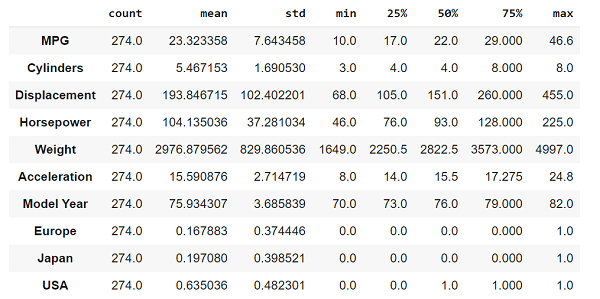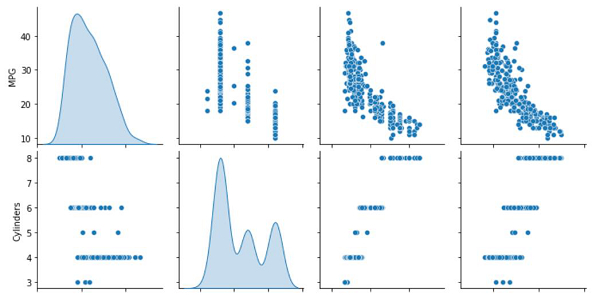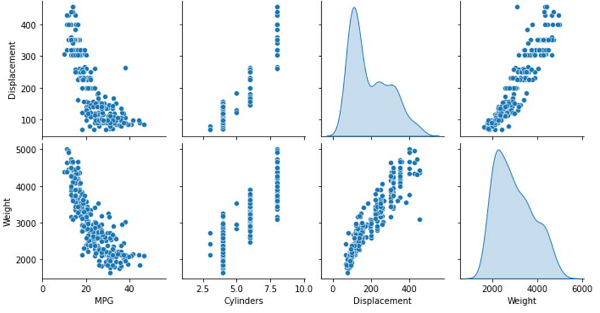# How can data be split and inspected to predict the fuel efficiency with Auto MPG dataset using TensorFlow?

PythonServer Side ProgrammingProgramming

Tensorflow is a machine learning framework that is provided by Google. It is an open−source framework used in conjunction with Python to implement algorithms, deep learning applications and much more. It is used in research and for production purposes. It has optimization techniques that help in performing complicated mathematical operations quickly. This is because it uses NumPy and multi-dimensional arrays. These multi-dimensional arrays are also known as ‘tensors’. The framework supports working with deep neural network. It is highly scalable, and comes with many popular datasets.

Tensor is a data structure used in TensorFlow. It helps connect edges in a flow diagram. This flow diagram is known as the ‘Data flow graph’. Tensors are nothing but multidimensional array or a list.

The aim behind a regression problem is to predict the output of a continuous or discrete variable, such as a price, probability, whether it would rain or not and so on.

The dataset we use is called the ‘Auto MPG’ dataset. It contains fuel efficiency of 1970s and 1980s automobiles. It includes attributes like weight, horsepower, displacement, and so on. With this, we need to predict the fuel efficiency of specific vehicles.

We are using the Google Colaboratory to run the below code. Google Colab or Colaboratory helps run Python code over the browser and requires zero configuration and free access to GPUs (Graphical Processing Units). Colaboratory has been built on top of Jupyter Notebook.

Following is the code snippet wherein we will see how can data be split and inspected to predict the fuel efficiency with Auto MPG dataset using TensorFlow −

## Example

print("Splitting the training and testing dataset")
train_dataset = dataset.sample(frac=0.7, random_state=0)
test_dataset = dataset.drop(train_dataset.index)

print("Plotting the training data as a visualization")
sns.pairplot(train_dataset[['MPG', 'Cylinders', 'Displacement', 'Weight']], diag_kind='kde')

print("Understanding the statistics associated with the data")
train_dataset.describe().transpose()

## Output

Splitting the training and testing dataset
Plotting the training data as a visualization
Understanding the statistics associated with the data## Explanation

• Once the data has been cleaned, the data is split into training and test dataset.

• 70 percent of the data is used for training and the remaining 30 percent is used for testing.

• This training data is visualized on the console using the seaborn package.

• The statistics of the data, such as count, mean, median, and so on is displayed using the ‘describe’ function.